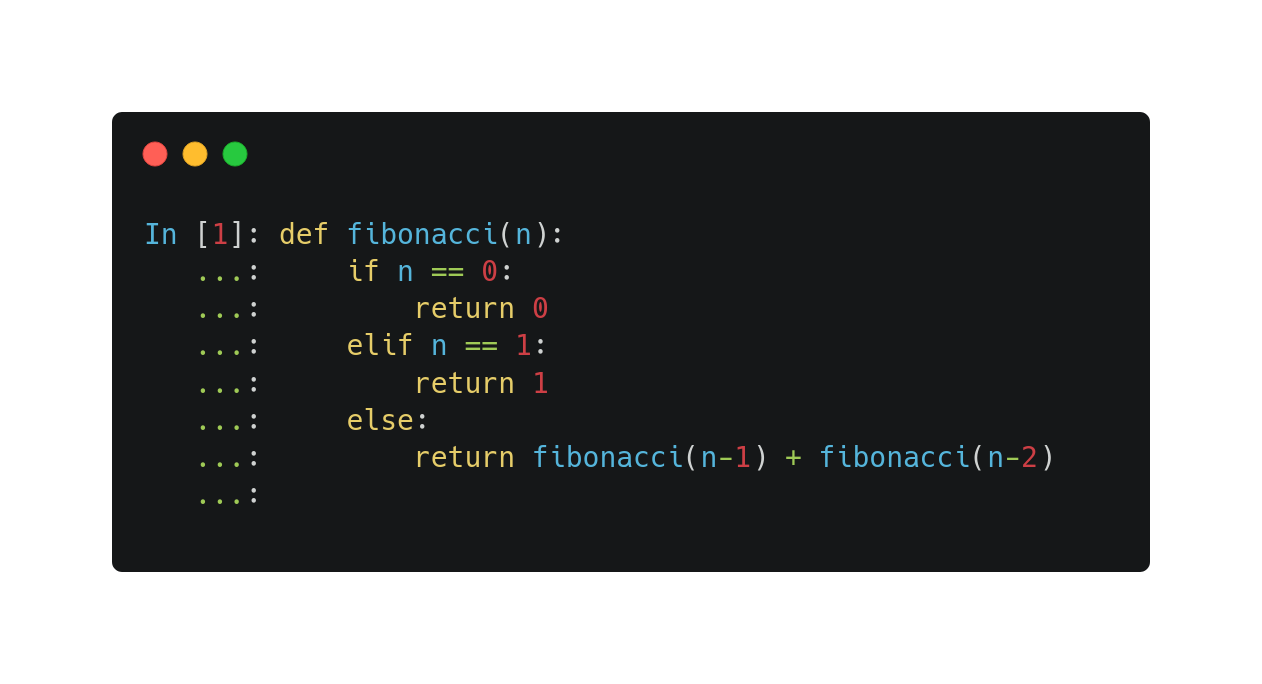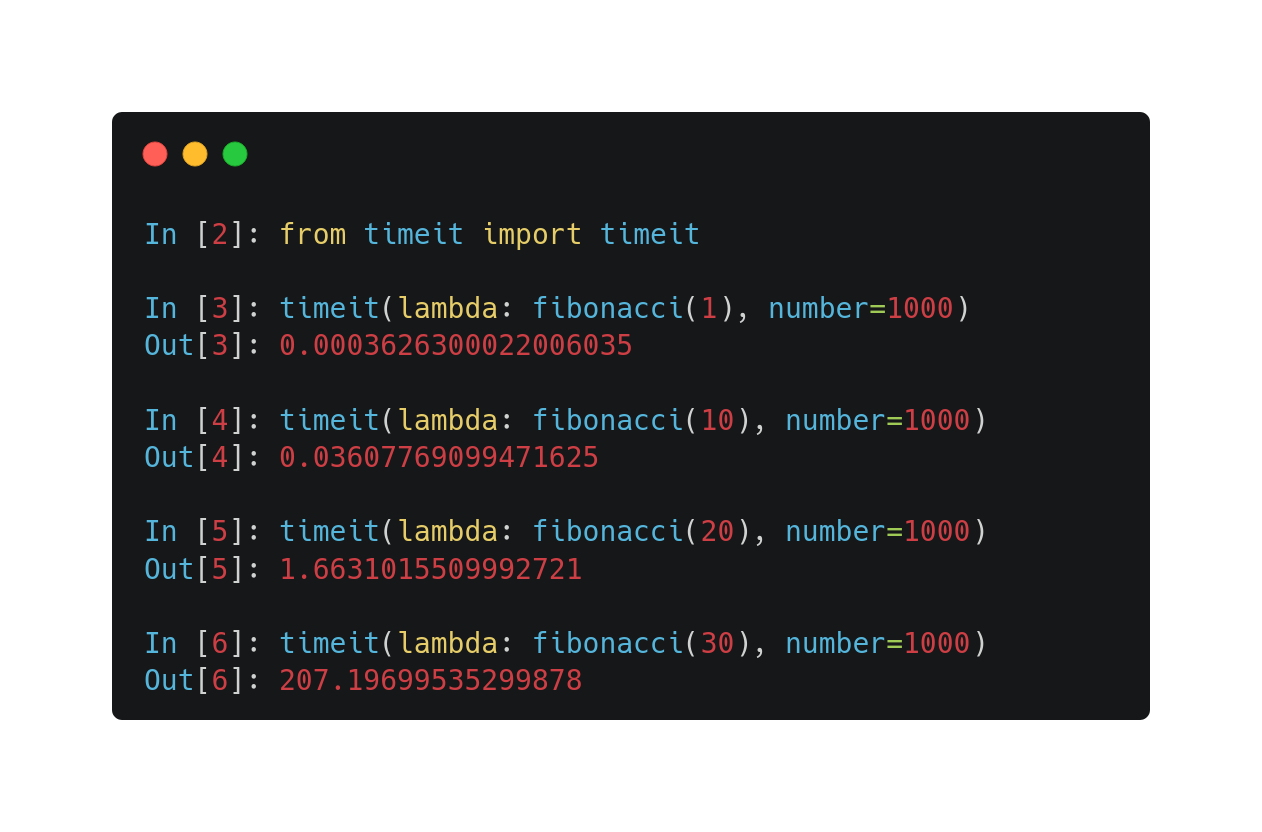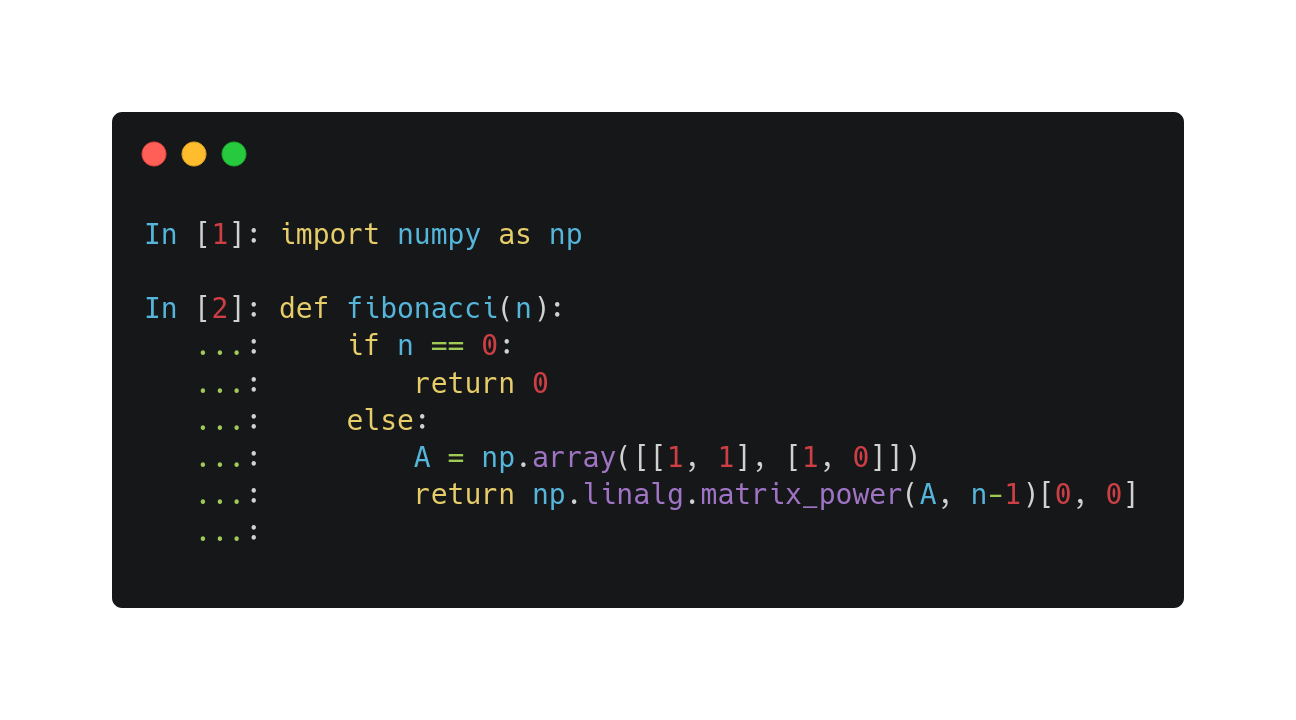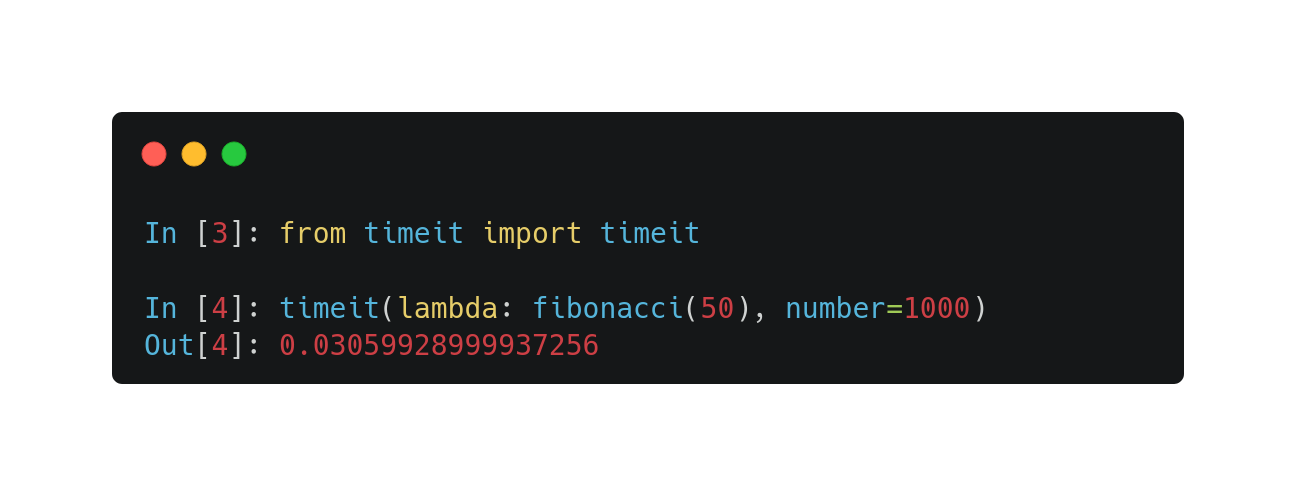# Recursion with matrix multiplicationThere is a mind-blowing application of matrix multiplication: doing recursion (almost) at the speed of light!

By the end of this post, you'll learn precisely how. Trust me, if you are into programming and math, you want to know this trick.

Let's start with the simplest example for recursion: Fibonacci numbers. Each Fibonacci number is the sum of the previous one and the one before. The recursion starts with $\textstyle 0$ and $\textstyle 1$.

\begin{align*} F_0 &= 1 \\ F_1 &= 1 \\ F_n &= F_{n-1} + F_{n-2} \end{align*}

In Python, the implementation is rather straightforward.Can you guess the issue? The execution is extremely slow, as each function call involves two more calls. Thus, the fibonacci(n) calls itself many times!

If we measure the execution, we find that the time increases exponentially with n.Is there any way to improve this? Yes. Spoiler alert: it's all about matrix multiplication.

## Matrix multiplication from another perspective

Now, let's talk about matrix multiplication. What do you get when multiplying a row and a column vector? Their inner product.

$\begin{bmatrix} a & b \end{bmatrix} \begin{bmatrix} x \\ y \end{bmatrix} = ax + by$

How does this relate to the Fibonacci numbers? Simple: we can express the Fibonacci recursion in terms of vectors.

$\begin{bmatrix} F_n & F_{n-1} \end{bmatrix} \begin{bmatrix} 1 \\ 1 \end{bmatrix} = F_n + F_{n-1} = F_{n + 1}$

With one small trick, we can turn this into an iterative process. By adding a second column to the right side, we can copy the $\textstyle n$-th Fibonacci number over. Thus, we have a recursive relation:

\begin{align*} \begin{bmatrix} F_n & F_{n-1} \end{bmatrix} \begin{bmatrix} 1 & 1 \\ 1 & 0 \end{bmatrix} &= \begin{bmatrix} F_n + F_{n-1} & F_n \end{bmatrix} \\ &= \begin{bmatrix} F_{n+1} & F_{n} \end{bmatrix}. \end{align*}

We can express the above without recursion! Applying our matrix recursion n times, we obtain an explicit formula to calculate the Fibonacci numbers:

$\begin{bmatrix} F_n & F_{n-1} \end{bmatrix} \begin{bmatrix} 1 & 1 \\ 1 & 0 \end{bmatrix}^n = \begin{bmatrix} F_{n+1} & F_n \end{bmatrix}.$

This is much faster to compute.

Just one more step!

By noticing that the Fibonacci numbers start with $0, 1, 1$, we can stack two shifted vectors on top of itself and obtain the $n+1$-th, $\textstyle n$-th, $n-1$-th Fibonacci numbers purely by raising the right matrix to the $\textstyle n$-th power:

\begin{align*} \begin{bmatrix} F_2 & F_1 \\ F_1 & F_0 \end{bmatrix} \begin{bmatrix} 1 & 1 \\ 1 & 0 \end{bmatrix}^{n-1} &= \begin{bmatrix} 1 & 1 \\ 1 & 0 \end{bmatrix}^n \\ &= \begin{bmatrix} F_{n+1} & F_n \\ F_n & F_{n-1} \end{bmatrix}. \end{align*}

By clearing everything up, we obtain an extremely elegant formula:

$\begin{bmatrix} 1 & 1 \\ 1 & 0 \end{bmatrix}^n = \begin{bmatrix} F_{n+1} & F_n \\ F_n & F_{n-1} \end{bmatrix}.$

Tell me it's not beautiful. I dare you.

We can quickly implement the formula in NumPy.Let's see how it performs!As you can see, it is much faster than the vanilla version. Moreover, while the vanilla implementation has exponential time complexity, this has linear. Quite the difference!

A small caveat, though. Due to integer overflow, NumPy is not suitable for this task.

Homework for you: write a plain Python implementation that takes advantage of the arbitrarily large ints!